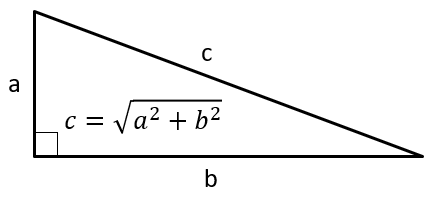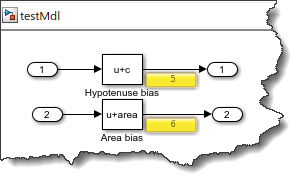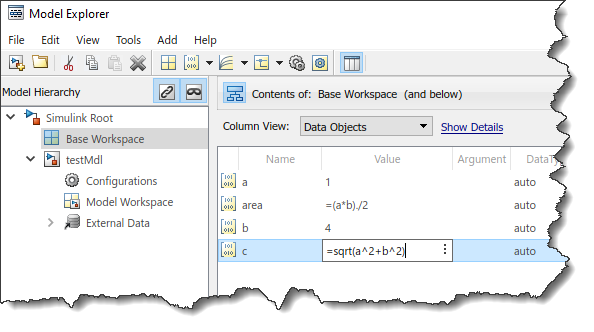# Specifying Variable Values Using Mathematical Expressions

Today I want to talk about variables, needed by a Simulink model, that are function of other variables.
Let's take the simple example of a right triangle:For most Simulink users, if you have a model that requires the length of the hypotenuse or the area of this triangle, you will probably end up writing a script like the following:
a = 3;
b = 4;
c = sqrt(a^2+b^2);
area = (a*b)/2;
Then, in block parameters, you will use variables c and area:Unrelated side notes regarding the above screenshot:
• I like to use the Bias block for this type of simple example; it receives a zero from the Inport block and adds the parameter value to it. This is only for experimenting/debugging, do not use this pattern instead of a Constant block in "normal" models.
• The yellow rectangles showing the Bias blocks output values are Port Value Displays. Those are useful to debug simulation results without adding blocks to the model.
While the above script does the job, it can lead to obvious issues. For example, what if I change variable a, re-evaluate the hypotenuse, but forget to re-evaluate the area?
While this is relatively easy to avoid for normal mode simulation, this can be easier to make mistakes when doing more advanced maneuvers, for example when using Fast Restart or Simulink Compiler.
The good thing is that there is a function that can help with that: slexpr

#### Using an Expression as Value of a Parameter Object

To create a tighter link between the original variables (a and b) and the variables that are functions of those (c and area), the first thing you need to do is convert those variables to Simulink.Parameter objects.
If you are not familiar with parameter objects, you can read more on that topic here. Those objects enable a lot more than what I describe in this post, especially regarding how C/C++ code is generated by Simulink Coder and Embedded Coder.
Once a and b have been created as parameter objects, you can use them with slexpr to specify an expression as value of other Simulink.Parameter objects:
You can then use variables c and area in a Simulink model just like if they where double data type variables as in the example at the beginning of this post:In order to compare the results from this original use case with examples in the following sections down this post, let's simulate the model programmatically and look at the result for the first Outport block:
out = sim("testMdl");
out.yout{1}.Values.Data
ans = 5
What becomes interesting is that once this is set up, you can simply change the value of original variables, a or b, and variables using it in an expression will automatically see the change.
a.Value = 8;
out = sim("testMdl");
out.yout{1}.Values.Data
ans = 8.9443

#### Leveraging Expressions in Fast Restart

This feature is especially useful for use cases like Fast Restart and Simulink Compiler, where the model gets compiled once and new variable values need to be explicitly passed to the sim command. Here is an example using Fast Restart:
a.Value = 3; % Restore value or a from the initial example
for i = 1:length(in)
in(i) = in(i).setVariable('a',i);
end
out = sim(in,'UseFastRestart','on','ShowProgress','off');
[out.yout{1}.Values.Data]
ans = 1×5
4.1231 4.4721 5.0000 5.6569 6.4031

#### Rapid Accelerator and Simulink Compiler

Another context where expressions as parameter values is useful is Rapid Accelerator. For the same five simulations as in the previous example, I can simulate in Rapid Accelerator without triggering a rebuild with the following code:
for i = 1:length(in)
in(i) = in(i).setModelParameter('simulationmode','rapid');
in(i) = in(i).setModelParameter('rapidacceleratoruptodatecheck','off');
in(i) = in(i).setVariable('a',i);
end
### Building the rapid accelerator target for model: testMdl ### Successfully built the rapid accelerator target for model: testMdl Build Summary Top model rapid accelerator targets built: Model Action Rebuild Reason ======================================================================================== testMdl Code generated and compiled Code generation information file does not exist. 1 of 1 models built (0 models already up to date) Build duration: 0h 0m 10.199s
out = sim(in,'ShowProgress','off');
[out.yout{1}.Values.Data]
ans = 1×5
4.1231 4.4721 5.0000 5.6569 6.4031
Without slexpr, all the simulations would have seen the same value for parameter c.
Since Simulink Compiler relies on the Rapid Accelerator technology, this technique would also apply in that context.

#### Model Explorer and Data Dictionary

If you are defining your data using the Model Explorer or in a Data Dictionary, you can use the "=" operator to do the same as what slexpr allows in MATLAB scripts.